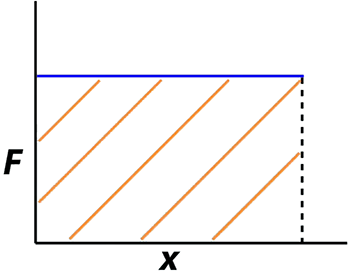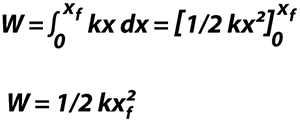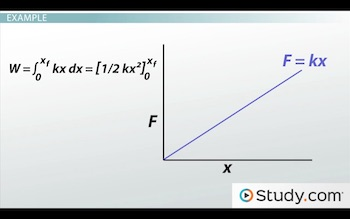# Work as an Integral

Lesson Transcript
Instructor: David Wood

David has taught Honors Physics, AP Physics, IB Physics and general science courses. He has a Masters in Education, and a Bachelors in Physics.

After watching this video, you will be able to solve calculus problems involving work and explain how that relates to the area under a force-displacement graph. A short quiz will follow.

## Work as an Integral

Work is an important quantity in physics. It's defined as the energy transferred from one type to another whenever a force is applied causing an object to move a distance. In equation form, work is a force multiplied by the displacement moved in the direction of that force. Or in other words, W = Fx cos (theta).

The cos (theta) part is a factor that just makes sure that the force you're looking at is in the right direction. It's the angle between the force and displacement vectors. So if you're pushing a block forwards, applying your force in the same direction as the displacement, theta is zero, and cos (0) = 1. So then work is just Fx. Or, if you're pushing down at an angle by putting in the angle between the direction, you're pushing and the direction you're moving, and you will get the component of your force in the direction it's moving multiplied by the displacement.

So far, none of this should be especially new. Work was introduced in another lesson in quite a bit of detail. But now, it's time to add a bit of calculus.In a basic sense, work is just force multiplied by displacement. Or, if you have a force-displacement graph, like the one shown, it would be the area under the graph. If the force is 20 newtons and the eventual displacement is 10 meters, then the work is 20 multiplied by 10, which is 200 Joules. The area of a rectangle is the product of each side, so it's the same as the area under the graph. But what if your force isn't a nice, constant 20 newtons? What if it's varying gradually? Or what if it's a curve?If the force is constantly changing, finding the area under the graph becomes more complex. And that's when you need a more fundamental equation for work. Work isn't just force multiplied by displacement. It's the integral of the force OVER the displacement. Or, in other words, if you have a force as a function of displacement and integrate it with respect to that displacement, you will get an expression for the work. Using the integral form shown, no matter how complex the shape of the force-distance graph, it will be possible to solve the problem.

An error occurred trying to load this video.

Try refreshing the page, or contact customer support.

Coming up next: Elliptical Orbits: Periods & Speeds

### You're on a roll. Keep up the good work!

Replay
Your next lesson will play in 10 seconds
• 0:02 Work as an Integral
• 2:09 Example
• 2:54 Practice Problems
• 4:18 Lesson Summary
Save Save

Want to watch this again later?

Timeline
Autoplay
Autoplay
Speed Speed

## ExampleIn this graph, the force is equal to kx - a constant times the displacement. That constant is just the slope of the graph. It's basically just a y = mx graph.

To find the work, we have to integrate this expression with respect to the force. So work equals the integral of kx dx, integrated from the initial displacement, 0, to the final displacement, xf. The integral of kx is ½ (kx)^2. And using the values of the limits, that comes out as W = ½ (kxf)^2. So that's our equation for the work done in this process. All we need is the slope of the graph and the final displacement, and we can calculate the work.

## Practice Problems

Time for some practice problems. Problem 1:

The force applied to an object is given by the equation F = 3x + 2. If the total displacement is 5 meters, how much work is done in this process?

To unlock this lesson you must be a Study.com Member.

### Register to view this lesson

Are you a student or a teacher?